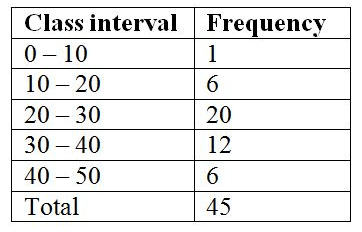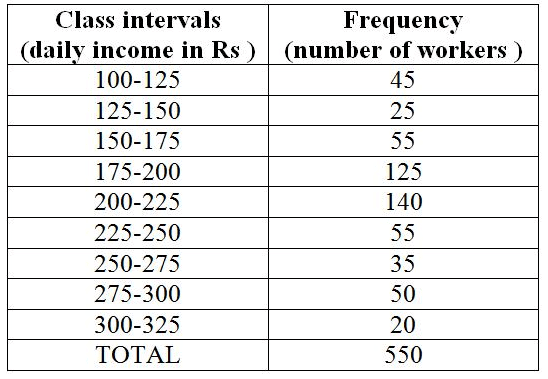Courses

# Test: Data Handling- 2

## 20 Questions MCQ Test Mathematics (Maths) Class 8 | Test: Data Handling- 2

Description
This mock test of Test: Data Handling- 2 for Class 8 helps you for every Class 8 entrance exam. This contains 20 Multiple Choice Questions for Class 8 Test: Data Handling- 2 (mcq) to study with solutions a complete question bank. The solved questions answers in this Test: Data Handling- 2 quiz give you a good mix of easy questions and tough questions. Class 8 students definitely take this Test: Data Handling- 2 exercise for a better result in the exam. You can find other Test: Data Handling- 2 extra questions, long questions & short questions for Class 8 on EduRev as well by searching above.
QUESTION: 1

### What is the upper limit of the class interval 30 – 40?Solution:

Upper limit is the highest number of the interval. Hence upper limit is 40 in the interval 30-40.

QUESTION: 2

Solution:
QUESTION: 3

### What is the size of the class interval?Solution:
QUESTION: 4

Which two classes have the same frequency?Solution:
QUESTION: 5

What is the lower limit of the class interval 40 – 50?Solution:

Lower limit is the least number of the interval. Hence 40 is the lowest limit.

QUESTION: 6

Which class has the highest frequency?Solution: Answer is A because it is asking Highest frequency and the highest frequency is 30 - 40
QUESTION: 7

When a die is thrown, total number of possible outcomes is ______.

Solution:
QUESTION: 8

When a coin is thrown, total number of possible outcomes is ______.

Solution:
QUESTION: 9

Two dice are thrown, find and number of outcomes.

Solution:

We know that in a single thrown of two die, the total number of possible outcomes is (6 × 6) = 36. Let S be the sample space. Then, n(S) = 36

QUESTION: 10

There are 2 Red, 3 Blue and 5 Black balls in a bag. A ball is drawn from the bag without looking in to the bag. What is the probability of getting a non-red ball?

Solution:

Non - red ball = 8
Probability = No of non red balls/Total no. of outcomes
= 8/10 = 4/5

QUESTION: 11

There are 2 Red, 3 Blue and 5 Black balls in a bag. A ball is drawn from the bag without looking in to the bag. What is the probability of getting a red ball?

Solution:
QUESTION: 12

There are 2 Red, 3 Blue and 5 Black balls in a bag. A ball is drawn from the bag without looking in to the bag. What is the probability of getting a blue ball?

Solution:
QUESTION: 13

There are 2 Red, 3 Blue and 5 Black balls in a bag. A ball is drawn from the bag without looking in to the bag. What is the probability of getting a black ball?

Solution:
QUESTION: 14

There are 2 Red, 3 Blue and 5 Black balls in a bag. A ball is drawn from the bag without looking in to the bag. What is the probability of getting a non-black ball?

Solution:
QUESTION: 15

There are 2 Red, 3 Blue and 5 Black balls in a bag. A ball is drawn from the bag without looking in to the bag. What is the probability of getting a non-blue ball?

Solution:
QUESTION: 16

What is the size of class intervals?Solution:
QUESTION: 17

Which class has the highest frequency?Solution:
QUESTION: 18

Which class has the lowest frequency?Solution:
QUESTION: 19

What is the frequency of class interval 300-325?Solution:
QUESTION: 20

What is the upper limit of the class interval 250-275?Solution: